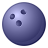# Route Length Issue

2868
5
11-15-2012 01:31 PMNew Contributor
Hello Guys,

I am using a "Network Analyst" tool in order to generate multiple shortest paths between about 1000 O-D pairs. When I run shortest path tool the first time, a first set of routes is calculated. Then rom the table of attributes I can obtain the length of each route. At the same time, I decided to "check" my lengths using "Calculate Geometry" tool and recalculate the same lengths. So, when I ran "Calculate Geometry" tool for the same routes I obtained lengths which were drastically different from the first set. Some of the largest differences are 7 km on a 16 km length. Thank you.

[ATTACH=CONFIG]19354[/ATTACH]
Tags (2)
5 Repliesby
Occasional Contributor III
hi,

Did you first export the routes layer to be a separate layer ? because routes layer is temporary and running calculate geometry may lead to misleading results. Also as i see, calculated length is always less than route length, so it suggests that calculate geometry may not be doing it for the whole route. just check again after exporting the routes to a permanent file.

regards,byEsri Regular Contributor
The route is computed by minimizing an impedance attribute and then returning the sum of that impedance.
What is the impedance attribute that you are using the solve the route? Is it based on the same length field that will be used when you calculate geometry? Do note that the calculate geometry is based on the current projection of the data. One way you can double check is to calculate geometry on the original street feature class and then compare it to the legnth impedance attribut you used. Are they the same? Most likely they will not be the same.

Another thing you can do is to run the first route pair, then use the GP tool (assuming you are using 10.1) CopySourceTraversedFeatures and it will generate a new line feature class from the route that shows all the individual streets and their length impedances.

Also I am a bit confused where you say you want to generate multiple paths between 1000 OD pairs and then you are using the route solver on the first set...are you trying to find all paths, that is 1000 by 1000 matrix? If yes, then you can use the OD Cost Matrix solver to do that.

Jay SandhubyEsri Regular Contributor
hi,

Did you first export the routes layer to be a separate layer ? because routes layer is temporary and running calculate geometry may lead to misleading results. Also as i see, calculated length is always less than route length, so it suggests that calculate geometry may not be doing it for the whole route. just check again after exporting the routes to a permanent file.

regards,

Routes may be "temporary"...but they are real features. They are in-memory. And you can run calculate geometry on them. If you know of any cases where you get misleading results then please let Esri tech support know so that we can fix such an issue. And calculate length cannot do partial feature lenghts. If it encounters problems then it should give some warning or error message.
Regards,
Jay SandhuNew Contributor
Hello Guys,

Here is clarification to some of your questions.
1) "Routes" layer was exported and then saved as a shape file. This is where I verified my lengths over again.
2) Impedance attribute is length and it is based on the same length field when I calculated geometry. Besides this, I had some linear cost restrictions at a factor 1.2.
3) I ran shortest path algorithm because I had to generate several alternatives between the same OD pairs. As a bigger task, I am building a utility function where I need to know the actual path plus several other viable options.
4) All data is based on the same projection. Even if we take curvature and refraction into account, that can not explain difference in lengths by a 7 km on a 20 km paths.

I verified several lengths and found that results obtained from "Calculate Geometry" tool are consistent with a "Measure Tool" (little ruler icon). I am very keen to know what length result is the actual length I can use in my further work.

Thank you.byEsri Regular Contributor
The route solver will sum the length of all the features (and prorated length of the partial features at begining and end of the route) and return the sum. It cannot be any different than the length field that is being used in the evaluator. Are you absolutely sure that the evaluator in the network dataset is pointing to the same length fields? You can use the network identify tool (second icon from right on the NA toolbar) to inspect the values of individual edge elements. Check if the values being returned are same as the length field that you have. ALSO, do you have some scaled cost barriers or turn delays or junction impedances, etc that could add to the overall length of the path?

If everything is ok, then you have to use the Copy Traversed Source Features tool to generate the lines, junctions and turns that make up the route. If it all looks ok, then I suggest you contact tech support for them to look at your data.

Jay Sandhu